## SPECTRUMHIST

 Creates either a spectrum or histogram of the colors in an image.

 USAGE: spectrumhist [-t type] [-s sort] [-w width] [-h height] [-m magnify] [-c colors] infile outfile USAGE: spectrumhist [-help] -t ... type ...... type of output; histogram (h) or spectrum (s); .................. default=histogram -s ... sort ...... sort mode; count (c), hue (h), sat (s), lum (l), .................. rgb (r) or bgr (b); default=hue -w ... width ..... width of bars in bar chart; integer>0; default=1 -h ... height .... height of output image; integer>0; default=100 -m ... magnify ... magnification for count scaling; integer>0; default=1 -c ... colors .... color reduction for input; 00. The default=1 -h height ... HEIGHT of the output image. Values are integer>0. The default=100 -m magnify ... MAGNIFY is the magnification factor for count scaling. This scales the height of each bar of the histogram, but does not affect the height of the image. Any bar that is taller than the image will be clipped. This is not relevant to type=spectrum. Values are integers>0. The default=1 -c colors ... COLORS is the number of colors desired for color reduction in the input image. Values are 0

### EXAMPLES

 Example 1 OriginalArguments: -t histogram -s hue -w 10 Arguments: -t histogram -s count -w 10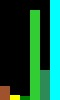Arguments: -t spectrum -s hue -w 10 Arguments: -t spectrum -s count -w 10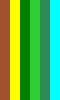Example 2Arguments: -t histogram -s hue Arguments: -t histogram -s countArguments: -t spectrum -s hue Arguments: -t spectrum -s countArguments: -t histogram -s hue -w 10 -c 24 Arguments: -t histogram -s count -w 10 -c 24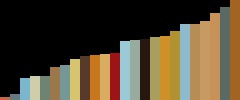Arguments: -t spectrum -s hue -w 10 -c 24 Arguments: -t spectrum -s count -w 10 -c 24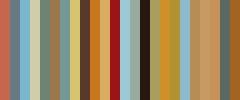Example 3Arguments: -t histogram -s hue -m 5 Arguments: -t histogram -s count -m 5Arguments: -t spectrum -s hue Arguments: -t spectrum -s countArguments: -t histogram -s hue -w 10 -c 12 Arguments: -t histogram -s count -w 10 -c 12Arguments: -t spectrum -s hue -w 10 -c 12 Arguments: -t spectrum -s count -w 10 -c 12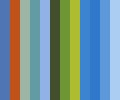Example 4 Original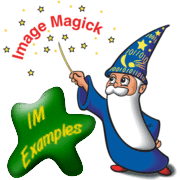Arguments: -t histogram -s hue -m 100 Arguments: -t histogram -s count -m 100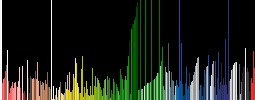Arguments: -t spectrum -s hue Arguments: -t spectrum -s count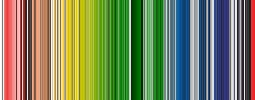Arguments: -t histogram -s hue -w 10 -c 12 Arguments: -t histogram -s count -w 10 -c 12Arguments: -t histogram -s hue -w 10 -c 12 -m 5 Arguments: -t histogram -s count -w 10 -c 12 -m 5Example 5 -- Spectrum vs SortArguments: -t spectrum -s count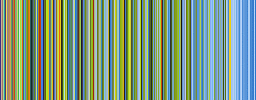Arguments: -t spectrum -s hue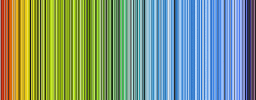Arguments: -t spectrum -s sat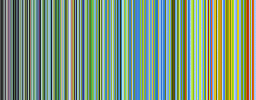Arguments: -t spectrum -s lumArguments: -t spectrum -s rgbArguments: -t spectrum -s bgrExample 6 -- Spectrum vs Sort OriginalArguments: -t spectrum -s count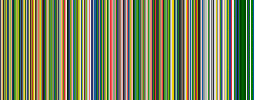Arguments: -t spectrum -s hueArguments: -t spectrum -s satArguments: -t spectrum -s lum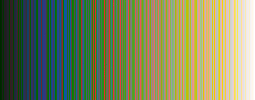Arguments: -t spectrum -s rgb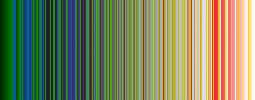Arguments: -t spectrum -s bgr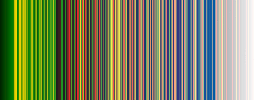What the script does is as follows for the case of type=histogram and sort=hue: Converts image to the desired number of colors and HSL color space Generates the histogram in text format and filters the counts and colors and sorts by increasing hue For each row of the histogram count and colors, appends the -size \${width}x\${ht} xc:hsl(hue,sat,bri) to a list, where ht=(magnify*100*count/maxcount Applies the list to a command to create each bar as an image and append them all together This is equivalent to the following IM commands convert -quiet -regard-warnings "\$infile" -alpha off +dither -colors \$colors -depth 8 +repage "\$tmpA1" ww=\$width hh=\$height string=`convert \$tmpA1 -colorspace HSL -format %c histogram:info:- |\ sed -n 's/^ *\(.*\):.*hsl(\(.*\))\$/\1 \2/p' | sort -n -k 2 |\ awk -v wd="\$width" -v mag="\$magnify" ' { list=""; i=NR; count[i]=\$1; color[i]=\$2; if (count[i]>maxcount) maxcount=count[i]; } END { for (i=1; i<=NR; i++) { ht[i]=int(mag*100*count[i]/maxcount+0.5); list=(list "\ " "-size\ " wd"x"ht[i] "\ " "xc:hsl("color[i])")" } {print list; } } '` convert -size \${ww}x\${hh} \$string -background black +append -flip \ -gravity south -crop \${www}x\${hh}+0+0 +repage \$outfile